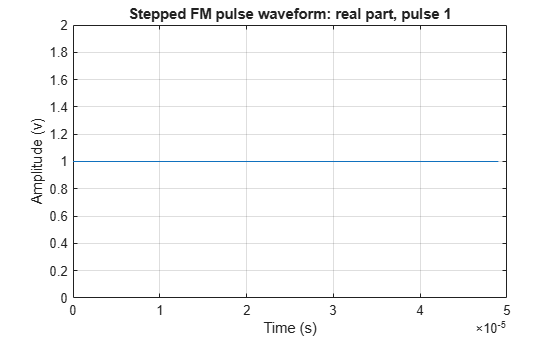Documentation

# plot

System object: phased.SteppedFMWaveform
Package: phased

Plot stepped FM pulse waveform

## Syntax

```plot(Hwav) plot(Hwav,Name,Value) plot(Hwav,Name,Value,LineSpec) h = plot(___) ```

## Description

`plot(Hwav)` plots the real part of the waveform specified by `Hwav`.

`plot(Hwav,Name,Value)` plots the waveform with additional options specified by one or more `Name,Value` pair arguments.

`plot(Hwav,Name,Value,LineSpec)` specifies the same line color, line style, or marker options as are available in the MATLAB® `plot` function.

`h = plot(___)` returns the line handle in the figure.

## Input Arguments

 `Hwav` Waveform object. This variable must be a scalar that represents a single waveform object. `LineSpec` Character vector to specifies the same line color, style, or marker options as are available in the MATLAB `plot` function. If you specify a `PlotType` value of `'complex'`, then `LineSpec` applies to both the real and imaginary subplots. Default: `'b'`

### Name-Value Pair Arguments

Specify optional comma-separated pairs of `Name,Value` arguments. `Name` is the argument name and `Value` is the corresponding value. `Name` must appear inside quotes. You can specify several name and value pair arguments in any order as `Name1,Value1,...,NameN,ValueN`.

 `'PlotType'` Specifies whether the function plots the real part, imaginary part, or both parts of the waveform. Valid values are `'real'`, `'imag'`, and `'complex'`. Default: `'real'` `'PulseIdx'` Index of the pulse to plot. This value must be a scalar. Default: `1`

## Output Arguments

 `h` Handle to the line or lines in the figure. For a `PlotType` value of `'complex'`, `h` is a column vector. The first and second elements of this vector are the handles to the lines in the real and imaginary subplots, respectively.

## Examples

expand all

Create and plot a stepped frequency pulse waveform.

``` waveform = phased.SteppedFMWaveform('NumSteps',3); plot(waveform);```